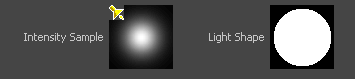# Spot Light Attributes

For a description of Maya’s Spot Light, see Create > Lights > Spot Light.

## Spot light Light Shape sample

The spotLightShape tab in the Attribute Editor also contains a Light Shape sample. This sample displays the shape the spot light casts and the light’s intensity. The following shows the default shape and intensity.Type

See Type.

Color

See Color.

Intensity

See Intensity.

Illuminates by Default
Emit Diffuse and Emit Specular
Decay Rate

See Decay Rate.

Cone Angle

The angle (in degrees) from edge to edge of the spot light’s beam. The valid range is 0.006 to 179.994. The default value is 40.

Penumbra Angle

The angle (in degrees) from the edge of the spot light’s beam over which the intensity of the spot light falls off linearly to zero. The valid range is -179.994 to 179.994. The slider range is -10 to 10. The default value is 0.

For example, if the Cone Angle value is 50 and the Penumbra Angle value is 10, the spot light has an effective spread of 70 (50 + 10 + 10) degrees; however, the spot light intensity decreases to 0 between the angles of 50 and 70 degrees. If the Cone Angle value is 50 and the Penumbra Angle value is 10, the spot light has an effective spread of 50 degrees and the spot light intensity decreases to 0 between the angles of 30 and 50 degrees.

Dropoff

Controls the rate at which light intensity decreases from the center to the edge of the spot light beam. The valid range is 0 to infinity. The slider range is 0 to 255.

Typical values are between 0 and 50. Values of 1 or less produce almost identical results (no discernible intensity decrease along the radius of the beam). The default value is 0 (no dropoff).Except where otherwise noted, this work is licensed under a Creative Commons Attribution-NonCommercial-ShareAlike 3.0 Unported License